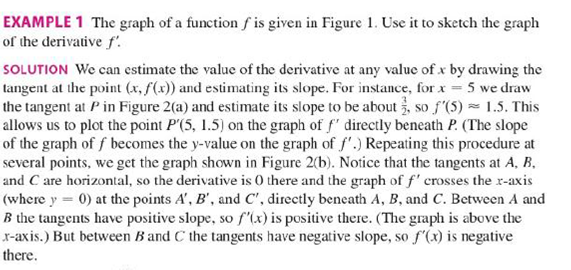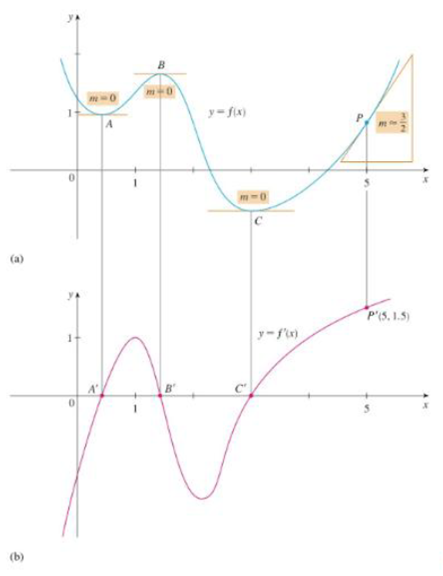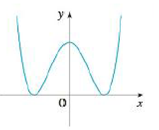Chapter 2.8, Problem 4E### Single Variable Calculus: Early Tr...

8th Edition
James Stewart
ISBN: 9781305270343

#### Solutions

Chapter
Section### Single Variable Calculus: Early Tr...

8th Edition
James Stewart
ISBN: 9781305270343
Textbook Problem

# Trace or copy the graph of the given function .f. (Assume that the axes have equal scales.) Then use the method of Example 1 to sketch the graph of f' below it.Example 1FIGURE 1FIGURE 2To determine

To sketch: The graph of derivative of f below the graph of f.

Explanation

From the given graph, it is observed that the graph of f contains the horizontal tangents at the three points. Let the three points be A, B and C.

Note that, the value of the derivative will be zero at the point where the function has the horizontal tangent.

Thus, the graph of f will be zero at the points A, B and C.

From the point A to left, the slope of the graph f is strictly negative which implies that the derivative graph f must have a negative functional value.

From the points between A and B, the slope of the graph f is strictly positive which implies that the derivative graph f must have a positive functional value.

And, the slope of the graph f between the points B and C is strictly negative, which implies that the derivative graph f must have a negative functional value

### Still sussing out bartleby?

Check out a sample textbook solution.

See a sample solution

#### The Solution to Your Study Problems

Bartleby provides explanations to thousands of textbook problems written by our experts, many with advanced degrees!

Get Started

#### In Exercises 914, evaluate the expression. 14. 3543183

Applied Calculus for the Managerial, Life, and Social Sciences: A Brief Approach

#### True or False: converges mean exists.

Study Guide for Stewart's Multivariable Calculus, 8th

#### True or False: is a convergent series.

Study Guide for Stewart's Single Variable Calculus: Early Transcendentals, 8th

Mathematics For Machine Technology